# equate Package Vignette

This vignette is an R markdown version of the following article:

Albano, A. D. (2016). equate: An R package for observed-score linking and equating. Journal of Statistical Software, 74(8), 1–36

# 1 Introduction

Equating is a statistical procedure commonly used in testing programs where administrations across more than one occasion and more than one examinee group can lead to overexposure of items, threatening the security of the test. In practice, item exposure can be limited by using alternate test forms; however, multiple forms lead to multiple score scales that measure the construct of interest at differing levels of difficulty. The goal of equating is to adjust for these differences in difficulty across alternate forms of a test, so as to produce comparable score scales.

Equating defines a functional statistical relationship between multiple test score distributions and thereby between multiple score scales. When the test forms have been created according to the same specifications and are similar in statistical characteristics, this functional relationship is referred to as an equating function, and it serves to translate scores from one scale directly to their equivalent values on another. The term linking refers to test forms which have not been created according to the same specifications, for example, forms which differ in length or content; in this case, the linked scales are considered similar but not interchangeable; they are related to one another via a linking function. Specific requirements for equating include equivalent constructs measured with equal reliability across test forms, equity in the equated results, symmetry of the equating function itself, and invariance of the function over examinee populations (for details, see Holland and Dorans 2006).

A handful of statistical packages are available for linking and equating test forms. Kolen and Brennan (2014) demonstrate a suite of free, standalone programs for observed-score and item response theory (IRT) linking and equating. Other packages, like equate, have been developed within the R environment Team (2020). For example, the R package kequate (Andersson, Bränberg, and Wiberg 2013) includes observed-score methods, but within a kernel equating framework. The R package plink (Weeks 2010) implements IRT linking under a variety of dichotomous, polytomous, unidimensional, and multidimensional models. The R package SNSequate (Burgos 2014) contains some functions for observed-score and kernel equating, along with IRT linking methods.

The equate package, available on the Comprehensive R Archive Network (CRAN) at https://CRAN.R-project.org/package=equate, is designed for observed-score linking and equating. It differs from other packages primarily in its overall structure and usability, its plotting and bootstrapping capabilities, and its inclusion of more recently developed equating and linking functions such as the general-linear, synthetic, and circle-arc functions, and traditional methods such as Levine, Tucker, and Braun/Holland. Equating with multiple anchor tests and external covariates is also supported, as demonstrated below. Linking and equating are performed using a simple interface, and plotting and summary methods are provided to facilitate the comparison of results and the examination of bootstrap and analytic equating error. Sample data and detailed help files are also included. These features make the package useful in teaching, research, and operational testing contexts.

This paper presents some basic linking and equating concepts and procedures. Equating designs are first discussed in Section 2. In Section 3, linear and nonlinear observed-score linking and equating functions are reviewed. In Section 4, methods are presented for linking and equating when examinee groups are not equivalent. Finally, in Section 5, the equate package is introduced and its basic functionality is demonstrated using three data sets.

# 2 Equating designs

Observed-score linking and equating procedures require data from multiple test administrations. An equating design specifies how the test forms and the individuals sampled to take them differ across administrations, if at all. For simplicity, in this paper and in the equate package, equating designs are categorized as either involving a single group, equivalent groups, or nonequivalent groups of examinees, and test forms are then constructed based on the type of group(s) sampled.

In the single-group design, one group, sampled from the target population $$T$$, takes two different test forms $$X$$ and $$Y$$, optionally with counterbalancing of administration orders (one group takes $$X$$ first, the other takes $$Y$$ first). Any differences in the score distributions on $$X$$ and $$Y$$ are attributed entirely to the test forms themselves, as group ability is assumed to be constant; thus, if the distributions are not the same, it is because the test forms differ in difficulty. Related to the single-group design is the equivalent-groups design, where one random sample from $$T$$ takes $$X$$ and another takes $$Y$$. Because the samples are taken randomly, group ability is again assumed to be constant, and any differences in the score distributions are again identified as form difficulty differences.

Without equivalent examinee groups, two related problems arise: 1) the target population must be defined indirectly using samples from two different examinee populations, $$P$$ and $$Q$$; and 2) the ability of these groups must then be accounted for, as ability differences will be a confounding factor in the estimation of form difficulty differences. In the nonequivalent-groups design these issues are both addressed through the use of what is referred to as an anchor test, $$V$$, a common measure of ability available for both groups. All non-equivalence in ability is assumed to be controlled or removed via this common measure. External covariates, such as scores from other tests, can also be used to control for group differences.

Equating procedures were initially developed using the single-group and equivalent-groups designs. In this simpler context, the traditional equating functions include mean, linear, and equipercentile equating; these and other equating functions are reviewed in Section 3. More complex procedures have been developed for use with the nonequivalent-groups design; these equating methods are presented in Section 4. Unless otherwise noted, additional details on the functions and methods described below can be found in Kolen and Brennan (2014).

# 3 Equating functions

Procedures for equating test forms to a common scale are referred to here and in the equate package as different types of equating functions. The equating function defines the equation for a line that expresses scores on one scale, or axis, in terms of the other. The available types of equating functions are categorized as straight-linear (i.e., linear), including identity, mean, and linear equating, and curvilinear (i.e., nonlinear), including equipercentile and circle-arc equating. The straight-line types differ from one another in intercept and slope, and the curvilinear lines differ in the number of coordinates on the line that are estimated, whether all of them or only one. Combinations of equating lines, referred to here as composite functions, are also discussed.

The goal of equating is to summarize the difficulty difference between $$X$$ to $$Y$$. As shown below, each equating type makes certain assumptions regarding this difference and how it does or does not change across the $$X$$ and $$Y$$ score scales. These assumptions are always expressed in the form of a line within the coordinate system for the $$X$$ and $$Y$$ scales.

## 3.1 Identity functions

Linear functions are appropriate when test form difficulties change linearly across the score scale, by a constant $$b$$ and rate of change $$a$$. Scores on $$X$$ are related to $$Y$$ as

$\begin{equation} y = ax + b. \tag{3.1} \end{equation}$

In the simplest application of Equation (3.1), the scales of $$X$$ and $$Y$$ define the line. Coordinates for scores of $$x$$ and $$y$$ are found based on their relative positions within each scale:

$\begin{equation} \frac{x - x_1}{x_2 - x_1} = \frac{y - y_1}{y_2 - y_1}. \tag{3.2} \end{equation}$

Here, $$(x_1, y_1)$$ and $$(x_2, y_2)$$ are coordinates for any two points on the line defined by the scales of $$X$$ and $$Y$$, for example, the minimum and maximum possible scale values. Solving Equation (3.2) for $$y$$ results in the identity linking function:

$\begin{equation} id_Y(x) = y = \frac{\Delta_Y}{\Delta_X}x + y_1 - \frac{\Delta_Y}{\Delta_X}x_1, \tag{3.3} \end{equation}$

where $$\Delta_Y = y_2 - y_1$$ and $$\Delta_X = x_2 - x_1$$,

$\begin{equation} a = \frac{\Delta_Y}{\Delta_X}, \tag{3.4} \end{equation}$

and

$\begin{equation} b = y_1 - \frac{\Delta_Y}{\Delta_X}x_1. \tag{3.5} \end{equation}$

The intercept $$b$$ can also be defined using the slope $$a$$ and any pair of $$X$$ and $$Y$$ coordinates $$(x_j, y_k)$$:

$\begin{equation} b = y_k - ax_j, \tag{3.6} \end{equation}$

where $$j = 1, 2, \dots, J$$ indexes the points on scale $$X$$ and $$k = 1, 2, \dots, K$$ indexes the points on scale $$Y$$.

The identity linking function is then expressed as

$\begin{equation} id_Y(x) = \frac{\Delta_Y}{\Delta_X}x + y_k - \frac{\Delta_Y}{\Delta_X}x_j. \tag{3.7} \end{equation}$

When the scales of $$X$$ and $$Y$$ are the same, $$a = 1$$ and $$b = 0$$, and Equation (3.7) reduces to the identity equating function:

$\begin{equation} ide_Y(x) = x. \tag{3.8} \end{equation}$

## 3.2 Mean functions

In mean linking and equating, form difficulty differences are estimated by the mean difference $$\mu_Y - \mu_X$$. Equation (3.7) is used to define a line that passes through the means of $$X$$ and $$Y$$, rather than the point $$(x_j, y_k)$$. The intercept from Equation (3.6) is expressed as

$\begin{equation} b = \mu_Y - a\mu_X. \tag{3.9} \end{equation}$

The mean linking function is then

$\begin{equation} mean_Y(x) =ax + \mu_Y - a\mu_X, \tag{3.10} \end{equation}$

where $$a$$ is found using Equation (3.4). When the scales of $$X$$ and $$Y$$ are the same, the slope $$a$$ is 1, which leads to the mean equating function:

$\begin{equation} meane_Y(x) = x + \mu_Y - \mu_X. \tag{3.11} \end{equation}$

In mean equating, coordinates for the line are based on deviation scores:

$\begin{equation} x - \mu_X = y - \mu_Y. \tag{3.12} \end{equation}$

In mean linking, coordinates are based on deviation scores relative to the scales of $$X$$ and $$Y$$:

$\begin{equation} \frac{x - \mu_X}{\Delta_X} = \frac{y - \mu_Y}{\Delta_Y}. \tag{3.13} \end{equation}$

## 3.3 Linear functions

The linear linking and equating functions also assume that the difficulty difference between $$X$$ and $$Y$$ changes by a constant amount $$a$$ across the score scale. However, in linear equating the slope is estimated using the standard deviations of $$X$$ and $$Y$$ as

$\begin{equation} a = \frac{\sigma_Y}{\sigma_X}. \tag{3.14} \end{equation}$

The linear linking and equating functions are defined as

$\begin{equation} lin_Y(x) = \frac{\sigma_Y}{\sigma_X}x + \mu_Y - \frac{\sigma_Y}{\sigma_X}\mu_X. \tag{3.15} \end{equation}$

In both the linear linking and equating functions, coordinates for the line are based on standardized deviation scores:

$\begin{equation} \frac{x - \mu_X}{\sigma_X} = \frac{y - \mu_Y}{\sigma_Y}. \tag{3.16} \end{equation}$

## 3.4 General linear functions

The identity, mean, and linear linking and equating functions presented above can all be obtained as variations of a general linear function $$glin_Y(x)$$ (Albano 2015). The general linear function is defined based on Equation (3.1) as

$\begin{equation} glin_Y(x) = \frac{\alpha_Y}{\alpha_X}x + \beta_Y - \frac{\alpha_Y}{\alpha_X}\beta_X, \tag{3.17} \end{equation}$

where

$\begin{equation} a = \frac{\alpha_Y}{\alpha_X} \tag{3.18} \end{equation}$

and

$\begin{equation} b = \beta_Y - \frac{\alpha_Y}{\alpha_X}\beta_X. \tag{3.19} \end{equation}$

Here, $$\alpha$$ is a general scaling parameter that can be estimated using $$\sigma$$, $$\Delta$$, another fixed value, or weighted combinations of these values. $$\beta$$ is a general centrality parameter that can be estimated using $$\mu$$, $$x_j$$ or $$y_k$$, other values, or weighted combinations of these values. Applications of the general linear function are discussed below and in Albano (2015).

## 3.5 Equipercentile functions

Equipercentile linking and equating define a nonlinear relationship between score scales by setting equal the cumulative distribution functions for $$X$$ and $$Y$$: $$F(x) = G(y)$$. Solving for $$y$$ produces the equipercentile linking function:

$\begin{equation} equip_Y(x) = G^{-1}[F(x)], \tag{3.20} \end{equation}$

which is also the equipercentile equating function $$equipe_Y(x)$$. When the score scales are discrete, which is often the case, the cumulative distribution function can be approximated using percentile ranks. This is a simple approach to continuizing the discrete score distributions (for details, see Kolen and Brennan 2014 ch. 2). Kernel equating, using Gaussian kernels, offers a more flexible approach to continuization (von Davier, Holland, and Thayer 2004), but differences between the methods tend to be negligible. The percentile rank method is currently used in the equate package. The equipercentile equivalent of a form-$$X$$ score on the $$Y$$ scale is calculated by finding the percentile rank in $$X$$ of a particular score, and then finding the form-$$Y$$ score associated with that form-$$Y$$ percentile rank.

Equipercentile equating is appropriate when $$X$$ and $$Y$$ differ nonlinearly in difficulty, that is, when difficulty differences fluctuate across the score scale, potentially at each score point. Each coordinate on the equipercentile curve is estimated using information from the distributions of $$X$$ and $$Y$$. Thus, compared to identity, mean, and linear equating, equipercentile equating is more susceptible to sampling error because it involves the estimation of as many parameters as there are unique score points on $$X$$.

Smoothing methods are typically used to reduce irregularities due to sampling error in either the score distributions or the equipercentile equating function itself. Two commonly used smoothing methods include polynomial loglinear presmoothing (Holland and Thayer 2000) and cubic-spline postsmoothing (Kolen 1984). The equate package currently supports loglinear presmoothing via the glm function. Details are provided below.

## 3.6 Circle-arc functions

Circle-arc linking and equating (Livingston and Kim 2009) also define a nonlinear relationship between score scales; however, they utilize only three score points in $$X$$ and $$Y$$ to do so: the low and high points, as defined above for the identity function, and a midpoint $$(x_j, y_k)$$. On their own, the low and high points define the identity linking function $$id_Y(x)$$, a straight line. When $$(x_j, y_k)$$ does not fall on the identity linking line, it can be connected to $$(x_1, y_1)$$ and $$(x_2, y_2)$$ by the circumference of a circle with center $$(x_c, y_c)$$ and radius $$r$$.

There are multiple ways of solving for $$(x_c, y_c)$$ and $$r$$ based on the three known points $$(x_1, y_1)$$, $$(x_j, y_k)$$, and $$(x_2, y_2)$$. For example, the center coordinates can be found by solving the following system of equations:

\begin{align} (x_1 - x_c)^2 + (y_1 - y_c)^2 &= r^2 \tag{3.21} \\ (x_j - x_c)^2 + (y_k - y_c)^2 &= r^2 \tag{3.22} \\ (x_2 - x_c)^2 + (y_2 - y_c)^2 &= r^2. \tag{3.23} \end{align}

Subtracting Equation (3.23) from (3.21) and (3.22) and rearranging terms leads to the following linear system:

\begin{align} 2(x_1 - x_2)x_c + 2(y_1 - y_2)y_c &= x_1^2 - x_2^2 + y_1^2 - y_2^2 \tag{3.24} \\ 2(x_j - x_2)x_c + 2(y_k - y_2)y_c &= x_j^2 - x_2^2 + y_k^2 - y_2^2. \tag{3.25} \end{align}

The center coordinates can then be obtained by plugging in the known values for $$(x_1, y_1)$$, $$(x_j, y_k)$$, and $$(x_2, y_2)$$ and again combining equations. The center and any other coordinate pair, e.g., $$(x_1, y_1)$$, are then used to find the radius:

$\begin{equation} r = \sqrt{(x_c - x_1)^2 + (y_c - y_1)^2}. \tag{3.26} \end{equation}$

Finally, solving Equation (3.26) for $$y$$ results in the circle-arc linking function:

$\begin{equation} circ_Y(x) = y_c \pm \sqrt{r^2 - (x - x_c)^2}, \tag{3.27} \end{equation}$

where the second quantity, under the square root, is added to $$y_c$$ when $$y_k > id_Y(x_j)$$ and subtracted when $$y_k < id_Y(x_j)$$. The circle-arc equating function $$circe_Y(x)$$ is obtained by using $$ide_Y(x_j)$$ in place of $$id_Y(x_j)$$ above.

Livingston and Kim (2010) refer to the circle connecting $$(x_1, y_1)$$, $$(x_j, y_k)$$, and $$(x_2, y_2)$$ as symmetric circle-arc equating. They also present a simplified approach, where the circle-arc function is decomposed into the linear component defined by $$(x_1, y_1)$$ and $$(x_2, y_2)$$, which is the identity function, and the circle defined by the points $$(x_1, y_1 - id_Y(x_1))$$, $$(x_j, y_k - id_Y(x_j))$$, and $$(x_2, y_2 - id_Y(x_2))$$. These low and high points reduce to $$(x_1, 0)$$ and $$(x_2, 0)$$, and the center coordinates can then be found as

$\begin{equation} x^*_c = \frac{(x_2^2-x_1^2)}{2(x_2-x_1)}, \tag{3.28} \end{equation}$

and

$\begin{equation} y^*_c = \frac{(x_1^2)(x_2 - x_j) - (x_j^2+y_k^{*2})(x_2 - x_1) + (x_2^2)(x_j - x_1)}{2[y_k^{*}(x_1 - x_2)]}, \tag{3.29} \end{equation}$

where $$y_k^{*} = y_k - id_Y(x_j)$$. Equation (3.26) is used to find the radius. Then, the simplified circle-arc function is the combination of the resulting circle-arc $$circ^{*}_Y(x)$$ and the identity function:

$\begin{equation} scirc_Y(x) = circ^*_Y(x) + id_Y(x). \tag{3.30} \end{equation}$

## 3.7 Composite functions

The circle-arc linking and equating functions involve a curvilinear combination of the identity and mean functions, where the circle-arc overlaps with the identity function at the low and high points, and with the mean function at the midpoint $$(\mu_X, \mu_Y)$$. A circle then defines the coordinates that connect these three points. This is a unique example of what is referred to here as a composite function.

The composite linking function is the weighted combination of any linear and/or nonlinear linking or equating functions:

$\begin{equation} comp_Y(x) = \displaystyle\sum_{h}w_{h}link_{hY}(x), \tag{3.31} \end{equation}$

where $$w_h$$ is a weight specifying the influence of function $$link_{hY}(x)$$ in determining the composite.

Equation (3.31) is referred to as a linking function, rather than an equating function, because it will typically not meet the symmetry requirement of equating. For symmetry to hold, the inverse of the function that links $$X$$ to $$Y$$ must be the same as the function that links $$Y$$ to $$X$$, that is, $$comp_Y^{-1}(x) = comp_X(y)$$, which is generally not true when using Equation (3.31). Holland and Strawderman (2011) show how symmetry can be maintained for any combination of two or more linear functions. The weighting system must be adjusted by the slopes for the linear functions being combined, where the adjusted weight $$W_h$$ is found as

$\begin{equation} W_h = \frac{w_h(1 + a_h^p)^{-1/p}}{\displaystyle\sum_hw_h(1 + a_h^p)^{-1/p}}. \tag{3.32} \end{equation}$

Here, $$a_h$$ is the slope for a given linear function $$link_h$$, and $$p$$ specifies the type of $$L_p$$-circle with which symmetry is defined. For details, see Holland and Strawderman (2011).

# 4 Equating methods

The linking and equating functions presented above are defined in terms of a single target population $$T$$, and they are assumed to generalize to this population. A subscript, e.g., $$X_T$$, is omitted for simplicity; it is presumed that $$X = X_T$$ and $$Y = Y_T$$. In the nonequivalent-groups design, scores come from two distinct populations, referred to here as populations $$P$$ and $$Q$$. Because the target population is not directly sampled, the linking and equating functions are redefined in terms of a weighted combination of $$P$$ and $$Q$$, where $$T = w_PP + w_QQ$$ and $$w_P$$ and $$w_Q$$ are proportions that sum to 1. This mixture of $$P$$ and $$Q$$ is referred to as the synthetic population (Braun and Holland 1982).

Linear equating is presented for the synthetic population first. All of the means and standard deviations in Equation (3.15) are estimated as weighted combinations of estimates from $$P$$ and $$Q$$, where

$\begin{equation} \mu_{X} = w_P\mu_{X_P} + w_Q\mu_{X_Q}, \tag{4.1} \end{equation}$

$\begin{equation} \mu_{Y} = w_P\mu_{Y_P} + w_Q\mu_{Y_Q}, \tag{4.2} \end{equation}$

$\begin{equation} \sigma_{X}^2 = w_P\sigma_{X_P}^2 + w_Q\sigma_{X_Q}^2 + w_Pw_Q(\mu_{X_P} - \mu_{X_Q})^2, \tag{4.3} \end{equation}$

and

$\begin{equation} \sigma_{Y}^2 = w_P\sigma_{Y_P}^2 + w_Q\sigma_{Y_Q}^2 + w_Pw_Q(\mu_{Y_P} - \mu_{Y_Q})^2. \tag{4.4} \end{equation}$

Because $$X$$ is not administered to $$Q$$ and $$Y$$ is not administered to $$P$$, the terms $$\mu_{X_Q}$$, $$\mu_{Y_P}$$, $$\sigma_{X_Q}^2$$, and $$\sigma_{X_Q}^2$$ in Equations (4.1) through (4.4) are obtained using available information for $$X$$, $$Y$$, and the anchor test $$V$$. This results in the following synthetic parameter estimates (for details, see Kolen and Brennan 2014):

$\begin{equation} \mu_{X} = \mu_{X_P} - w_Q\gamma_P(\mu_{V_P} - \mu_{V_Q}), \tag{4.5} \end{equation}$

$\begin{equation} \mu_{Y} = \mu_{Y_Q} + w_P\gamma_Q(\mu_{V_P} - \mu_{V_Q}), \tag{4.6} \end{equation}$

$\begin{equation} \sigma_{X}^2 = \sigma_{X_P}^2 - w_Q\gamma_P^2(\sigma_{V_P}^2 - \sigma_{V_Q}^2) + w_Pw_Q\gamma_P^2(\mu_{V_P} - \mu_{V_Q})^2, \tag{4.7} \end{equation}$

and $\begin{equation} \sigma_{Y}^2 = \sigma_{Y_Q}^2 + w_P\gamma_Q^2(\sigma_{V_P}^2 - \sigma_{V_Q}^2) + w_Pw_Q\gamma_Q^2(\mu_{V_P} - \mu_{V_Q})^2. \tag{4.8} \end{equation}$

The $$\gamma$$ terms in Equations (4.5) through (4.8) represent the relationship between total scores on $$X$$ and $$Y$$ and the respective anchor scores on $$V$$. $$\gamma_P$$ and $$\gamma_Q$$ are used along with the weights to adjust the observed $$\mu$$ and $$\sigma^2$$ for $$X$$ and $$Y$$ in order to obtain corresponding estimates for the synthetic population. For example, when $$w_P = 0$$ and $$w_Q = 1$$, $$\mu_{Y} = \mu_{Y_Q}$$, and conversely $$\mu_{X_Q}$$ will be adjusted the maximum amount when obtaining $$\mu_{X}$$. The same would occur with the estimation of synthetic variances. Furthermore, the adjustments would be completely removed if populations $$P$$ and $$Q$$ did not differ in ability, where $$\mu_{V_P} = \mu_{V_Q}$$ and $$\sigma_{V_P}^2 = \sigma_{V_Q}^2$$.

A handful of techniques have been developed for estimating the linear $$\gamma$$ terms required by Equations (4.5) through (4.8), and the terms required for equipercentile equating, as described below. These techniques all make certain assumptions about the relationships between total scores and anchor scores for populations $$P$$ and $$Q$$. The techniques are referred to here as equating methods. The equate package supports the Tucker, nominal weights, Levine observed-score, Levine true-score, Braun/Holland, frequency estimation, and chained equating methods (although chained equating does not rely on $$\gamma$$, it does make assumptions about the relationship between total and anchor scores). The Tucker, nominal weights, Braun/Holland, and frequency estimation methods are also available for use with multiple anchor tests; see Appendix 8.1. Table 4.1 shows the supported methods that apply to each equating type.

## 4.1 Tucker

In Tucker equating (Levine 1955) the relationship between total and anchor test scores is defined in terms of regression slopes, where $$\gamma_P$$ is the slope resulting from the regression of $$X$$ on $$V$$ for population $$P$$, and $$\gamma_Q$$ the slope from a regression of $$Y$$ on $$V$$ for population $$Q$$:

$\begin{equation} \gamma_P = \frac{\sigma_{X_P,V_P}}{\sigma_{V_P}^2} \,\,\,\, \text{and} \,\,\,\, \gamma_Q = \frac{\sigma_{Y_Q,V_Q}}{\sigma_{V_Q}^2}. \tag{4.9} \end{equation}$

The Tucker method assumes that across populations: 1) the coefficients resulting from a regression of $$X$$ on $$V$$ are the same, and 2) the conditional variance of $$X$$ given $$V$$ is the same. These assumptions apply to the regression of $$Y$$ on $$V$$ and the covariance of $$Y$$ given $$V$$ as well. They also apply to the regression of $$X$$ or $$Y$$ on multiple anchor tests and external covariates (e.g., Angoff 1984); see Appendix 8.1.1 for details.

Table 4.1: Applicable equating types and methods.
type nominal tucker levine braun frequency chained
mean X X X X X
linear X X X X
general linear X X X X
equipercentile X X
circle-arc X X X X X
multiple anchors X X X X

## 4.2 Nominal weights

Nominal weights equating is a simplified version of the Tucker method where the total and anchor tests are assumed to have similar statistical properties and to correlate perfectly within populations $$P$$ and $$Q$$. In this case the $$\gamma$$ terms can be approximated by the ratios

$\begin{equation} \gamma_P = \frac{K_X}{K_V} \,\,\,\, \text{and} \,\,\,\, \gamma_Q = \frac{K_Y}{K_V}, \tag{4.10} \end{equation}$

where $$K$$ is the number of items on the test. See Babcock, Albano, and Raymond (2012) for a description and examples with a single anchor. When using multiple anchor tests, a $$\gamma$$ term is again estimated for each anchor test, as in the multi-anchor Tucker method; see Appendix 8.1.2.

## 4.3 Levine

Assumptions for the Levine (Levine 1955) observed-score method are stated in terms of true scores (though only observed scores are used), where, across both populations: 1) the correlation between true scores on $$X$$ and $$V$$ is 1, as is the correlation between true scores on $$Y$$ and $$V$$; 2) the coefficients resulting from a linear regression of true scores for $$X$$ on $$V$$ are the same, as with true scores for $$Y$$ on $$V$$; and 3) measurement error variance is the same (across populations) for $$X$$, $$Y$$, and $$V$$. These assumptions make possible the estimation of $$\gamma$$ as

$\begin{equation} \gamma_P = \frac{\sigma_{X_P}^2}{\sigma_{X_P,V_P}} \,\,\,\, \text{and} \,\,\,\, \gamma_Q = \frac{\sigma_{Y_Q}^2}{\sigma_{Y_Q,V_Q}}, \tag{4.11} \end{equation}$

which are the inverses of the respective regression slopes for $$V$$ on $$X$$ and $$V$$ on $$Y$$. The Levine true-score method is based on the same assumptions as the observed-score method; however, it uses a slightly different linear equating function in place of Equation (3.15):

$\begin{equation} lin_Y(x) = \frac{\gamma_Q}{\gamma_P}X(x - \mu_{X_P}) + \mu_{Y_Q} + \gamma_Q(\mu_{V_P} - \mu_{V_Q}), \end{equation}$

with $$\gamma$$ defined by Equation (4.11). Hanson (1991) and Kolen and Brennan (2014) provide justifications for using the Levine true-score method.

## 4.4 Frequency estimation

The frequency estimation or poststratification method is used in equipercentile equating under the nonequivalent-groups design. It is similar to the methods described above in that it involves a synthetic population. However, in this method full score distributions for the synthetic population taking forms $$X$$ and $$Y$$ are required. When the assumptions are made that 1) the conditional distribution of total scores on $$X$$ for a given score point in $$V$$ is the same across populations, and 2) the conditional distribution of total scores on $$Y$$ for a given score point in $$V$$ is the same across populations, the synthetic distributions can be obtained as

$\begin{equation} \text{Pr}(x) = w_P\text{Pr}_P(x) + w_Q\sum \text{Pr}_P(x|v)\text{Pr}_Q(v) \tag{4.12} \end{equation}$

and

$\begin{equation} \text{Pr}(y) = w_Q\text{Pr}_Q(y) + w_P\sum \text{Pr}_Q(y|v)\text{Pr}_P(v). \tag{4.13} \end{equation}$

Here, $$\text{Pr}(x)$$, $$\text{Pr}(y)$$, and $$\text{Pr}(v)$$ denote the distribution functions for forms $$X$$, $$Y$$, and $$V$$ respectively. Percentile ranks can be taken for the cumulative versions of Equations (4.12) and (4.13) to obtain Equation (3.20). The frequency estimation method can also accommodate multiple anchors and external covariates; see Appendix 8.1.3.

## 4.5 Braun/Holland

As a kind of extension of the frequency estimation method, the Braun/Holland method (Braun and Holland 1982) defines a linear function relating $$X$$ and $$Y$$ that is based on the means and standard deviations of the synthetic distributions obtained via frequency estimation. Thus the full synthetic distributions are estimated, as with frequency estimation, but only in order to obtain their means and standard deviations.

## 4.6 Chained

Finally, chained equating (Livingston, Dorans, and Wright 1990) can be applied to both linear and equipercentile equating under the nonequivalent-groups with anchor test design. The chained method differs from all other methods discussed here in that it does not explicitly reference a synthetic population. Instead, it introduces an additional equating function in the process of estimating score equivalents; see Appendix 8.2 for details. For both linear and equipercentile equating the steps are as follows:

1. Define the function relating $$X$$ to $$V$$ for population P, $$link_{V_P}(x)$$,
2. Define the function relating $$V$$ to $$Y$$ for population Q, $$link_{Y_Q}(v)$$,
3. Equate $$X$$ to the scale of $$Y$$ using both functions, where $$chain_Y(x) = link_{Y_Q}[link_{V_P}(x)]$$.

Chained methods are based on the assumptions that 1) the equating of $$X$$ to $$V$$ is the same for $$P$$ and $$Q$$, and 2) the equating of $$V$$ to $$Y$$ is the same for $$P$$ and $$Q$$.

## 4.7 Methods for the general linear function

The general linear equating function can be utilized with any combination of weighted means and standard deviations from Equations (4.1) through (4.4). Thus, any methods for nonequivalent groups that estimate means or means and standard deviations for the synthetic population can be implemented within the general linear function. In the equate package, these methods are currently Tucker, nominal-weights, Levine observed-score, and Braun/Holland. See Albano (2015) for examples. Composites of these methods can also be obtained.

## 4.8 Methods for the circle-arc function

As discussed above, the circle-arc equating function combines a linear with a curvilinear component based on three points in the $$X$$ and $$Y$$ score distributions. Although all three points can be obtained using the general linear function, the first and third of these points are typically determined by the score scale whereas the midpoint is estimated. Equating methods used with circle-arc equating in the nonequivalent-groups design apply only to estimation of this midpoint. Livingston and Kim (2009) demonstrated chained linear equating of means, under a nonequivalent-groups design. The midpoint could also be estimated using other linear methods, such as Tucker or Levine.

Note that circle-arc equating is defined here as an equating type, and equating methods are used to estimate the midpoint. When groups are considered equivalent (i.e., an anchor test is not used) equating at the midpoint is simply mean equating, as mentioned above (replace $$x$$ with $$\mu_X$$ in Equation (3.15) to see why this is the case). With scores on an anchor test, both Tucker and Levine equating at the midpoint also reduce to mean equating. However, chained linear equating at the midpoint differs from chained mean (see Appendix 8.2).

# 5 Using the equate package

## 5.1 Sample data

The equate package includes three sample data sets. The first, ACTmath, comes from two administrations of the ACT mathematics test, and is used throughout Kolen and Brennan (2014). The test scores are based on an equivalent-groups design and are contained in a three-column data frame where column one is the 40-point score scale and columns two and three are the number of examinees for $$X$$ and $$Y$$ obtaining each score point.

The second data set, KBneat, is also used in Kolen and Brennan (2014). It contains scores for two forms of a 36-item test administered under a nonequivalent-groups design. A 12-item anchor test is internal to the total test, where anchor scores contribute to an examinee’s total score. The number of non-anchor items that are unique to each form is 24, and the highest possible score is 36. KBneat contains a separate total and anchor score for each examinee. It is a list of length two where the list elements x and y each contain a two-column data frame of scores on the total test and scores on the anchor test.

The third data set, PISA, contains scored cognitive item response data from the 2009 administration of the Programme for International Assessment (PISA). Four data frames are included in PISA: PISA$students contains scores on the cognitive assessment items in math, reading, and science for all 5233 students in the USA cohort; PISA$booklets contains information about the structure of the test design, where multiple item sets, or clusters, were administered across 13 test booklets; PISA$items contains the cluster, subject, maximum possible score, item format, and number of response options for each item; and PISA$totals contains a list of cluster total scores for each booklet, calculated using PISA$students and PISA$booklets. For additional details, see the PISA help file which includes references to technical documentation.

## 5.2 Preparing score distributions

The equate package analyzes score distributions primarily as frequency table objects with class freqtab. For example, to equate the ACTmath forms, they must first be converted to frequency tables as follows.

library("equate")
act.x <- as.freqtab(ACTmath[, 1:2])
act.y <- as.freqtab(ACTmath[, c(1, 3)])

The freqtab class stores frequency distributions as table arrays, with a dimension for each of the variables included in the distribution. The function as.freqtab is used above because ACTmath already contains tabulated values; this code simply restructures the scales and counts for the two test forms and gives them the appropriate attributes. When printed to the console, freqtab objects are converted to data.frame. They are summarized with the summary method.

head(act.x)
#>   total count
#> 1     0     0
#> 2     1     1
#> 3     2     1
#> 4     3     3
#> 5     4     9
#> 6     5    18
rbind(x = summary(act.x), y = summary(act.y))
#>       mean       sd      skew     kurt min max    n
#> x 19.85239 8.212585 0.3751416 2.301379   1  40 4329
#> y 18.97977 8.940397 0.3525667 2.145331   1  40 4152

The constructor freqtab creates a frequency table from a vector or data frame of observed scores. With an anchor test, this becomes a bivariate frequency table. Bivariate distributions contain counts for all possible score combinations for the total and anchor scores. Multivariate distributions, e.g., containing scores on multiple anchor tests and external covariates, are also supported.

neat.x <- freqtab(KBneat$x, scales = list(0:36, 0:12)) neat.y <- freqtab(KBneat$y, scales = list(0:36, 0:12))

Finally, freqtab can also be used to sum scored item responses, and then tabulate the resulting total scores. In this case, the list items must contain vectors of the columns over which total scores should be calculated. For example, the following syntax creates a frequency table using four reading clusters from PISA booklet 6, with clusters R3 and R6 containing the unique items and clusters R5 and R7 containing the anchor items. The design argument is used to specify the single-group equating design, as the default when creating a bivariate frequency table is the nonequivalent-groups design.

attach(PISA)
r3items <- paste(items$itemid[items$clusterid == "r3a"])
r6items <- paste(items$itemid[items$clusterid == "r6"])
r5items <- paste(items$itemid[items$clusterid == "r5"])
r7items <- paste(items$itemid[items$clusterid == "r7"])
pisa <- freqtab(students[students$book == 6, ], items = list(c(r3items, r6items), c(r5items, r7items)), scales = list(0:31, 0:29), design = "sg") round(data.frame(summary(pisa), row.names = c("r3r6", "r5r7")), 2) #> mean sd skew kurt min max n #> r3r6 17.45 7.20 -0.18 2.01 1 31 396 #> r5r7 18.19 6.05 -0.65 2.72 1 29 396 A basic plot method is provided in the freqtab class. Univariate frequencies are plotted as vertical lines for the argument x, similar to a bar chart, and as superimposed curves for the argument y. When y is a matrix, each column of frequencies is added to the plot as a separate line. This feature is useful when examining smoothed frequency distributions, as demonstrated below. When x is a bivariate frequency table, a scatter plot with marginal frequency distributions is produced. See Figure 5.1 for an example of a univariate plot, and Figure 5.2 for an example of a bivariate plot. plot(x = act.x, lwd = 2, xlab = "Score", ylab = "Count")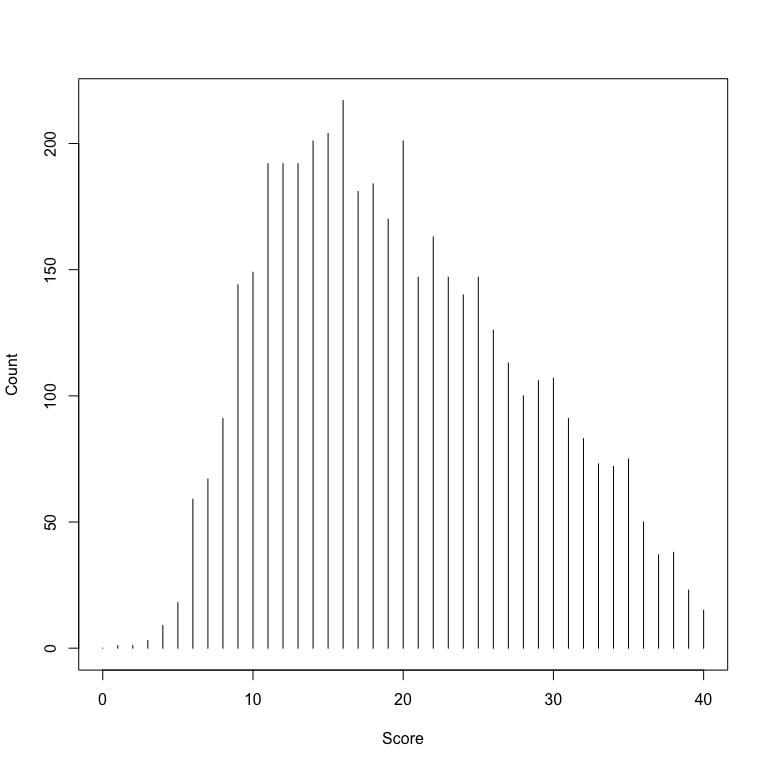Figure 5.1: Univariate plot of ACTmath total scores for form X. plot(neat.x)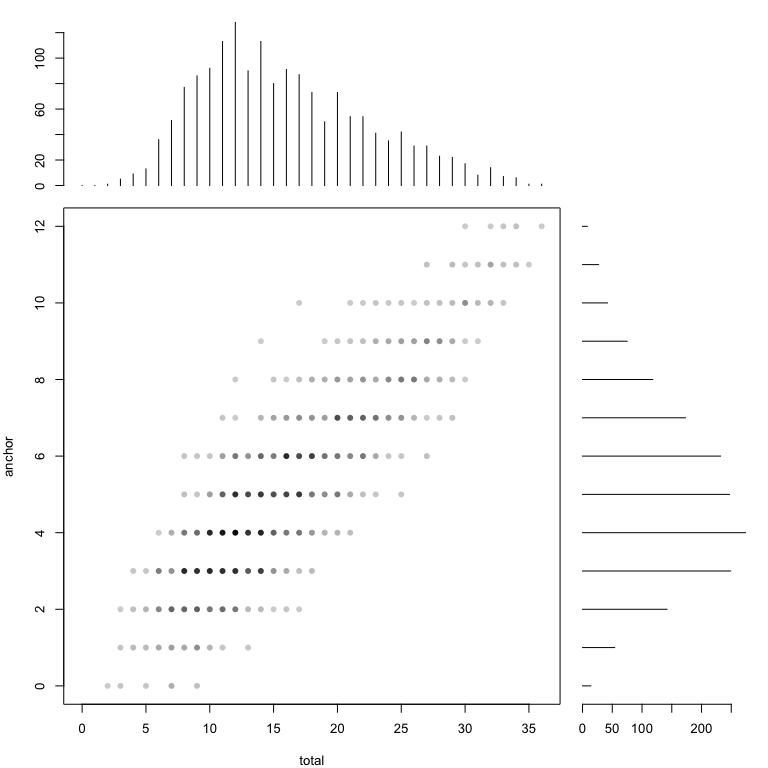Figure 5.2: Bivariate plot of KBneat total and anchor distributions. ## 5.3 Presmoothing The distributions in Figures 5.1 and 5.2 contain irregularities in their shapes that likely result, to some extent, from sampling error. The population distributions that these samples estimate are expected to be smoother, with less jaggedness between adjacent scores. Three methods are available for smoothing sample distributions with the objective of reducing these irregularities. The first, frequency averaging (Moses and Holland 2008) replaces scores falling below jmin with averages based on adjacent scores. This is implemented with smoothmethod = "average" in the presmoothing function. The second, adds a small relative frequency (again, jmin) to each score point while adjusting the probabilities to sum to one (as described by Kolen and Brennan 2014, 46). This is implemented using smoothmethod = "bump" in the presmoothing function. The third method of reducing irregularities in sample distributions is polynomial loglinear smoothing. Appendix 8.3 contains details on the model formula itself. In the equate package, loglinear models are fit using the presmoothing function with smoothmethod = "loglinear", which calls on the generalized linear model (glm) function in R. Models can be fit in three different ways. The first way is with a formula object, as follows. presmoothing(~ poly(total, 3, raw = T) + poly(anchor, 3, raw = T) + total:anchor, data = neat.x) This is similar to the approach used in other modeling functions in R, but with two restrictions: 1) the dependent variable, to the left of the ~, is set to be the score frequencies contained in data, and it does not need to be specified in the formula; and 2) the intercept is required and will be added if it has been explicitly removed in the formula. The formula smoothing method is efficient to write for simpler models, but it can be cumbersome for more complex models containing multiple interaction terms. The second way to specify the model is with a matrix of score functions (scorefun) similar to a model.matrix in R, where each column is a predictor variable in the model, as follows. neat.xsf <- with(as.data.frame(neat.x), cbind(total, total^2, total^3, anchor, anchor^2, anchor^3, total*anchor)) presmoothing(neat.x, smooth = "loglinear", scorefun = neat.xsf) The object neat.xsf is a matrix containing the total and anchor score scales to the first, second, and third powers, and the interaction between the two. The presmoothing results based on this score function are the same as those for the formula method above. One benefit of creating the score function externally is that it can be easily modified and used with other models. It can also include variables not contained within the raw frequency table, neat.x, in this example. The formula interface is limited in this respect, as the data} argument must be a frequency table, and it cannot include variables besides the total and anchor scores. The most efficient approach to specify a loglinear model in the presmoothing function is by including the degrees of the highest polynomial terms for each variable at each level of interaction. For example, in the formula and score function methods above, terms are included for both the total and anchor tests up to the third power and for the two-way interaction to the first power. This is specified compactly using degrees = list(c(3, 3), c(1, 1)), which can be reduced to degrees = list(3, 1). neat.xs <- presmoothing(neat.x, smooth = "log", degrees = list(3, 1)) This functionality extends to multivariate distributions. For example, three way interactions would be specified by including a third vector in the degrees list. For the bivariate example, the smoothed distributions in Figure 5.3 can be compared to the unsmoothed ones in Figure 5.2. Figure 5.4 superimposes the smoothed frequencies on the unsmoothed marginal distributions for a more detailed comparison of the different models. Descriptive statistics show that the smoothed distributions match the unsmoothed in the first three moments. neat.xsmat <- presmoothing(neat.x, smooth = "loglinear", degrees = list(3, 1), stepup = TRUE) plot(neat.xs)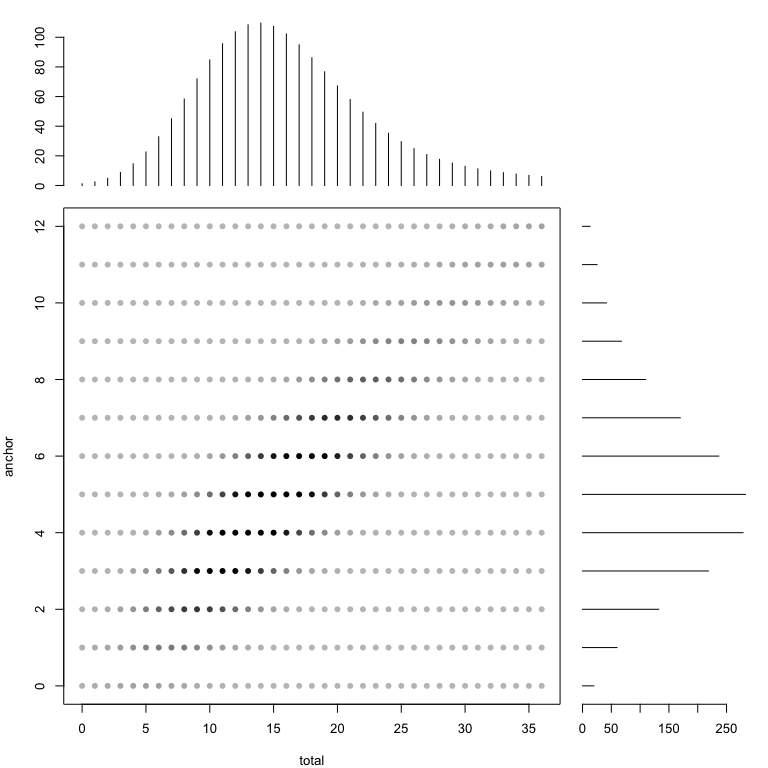Figure 5.3: Bivariate plot of smoothed KBneat total and anchor distributions. plot(neat.x, neat.xsmat, ylty = 1:5)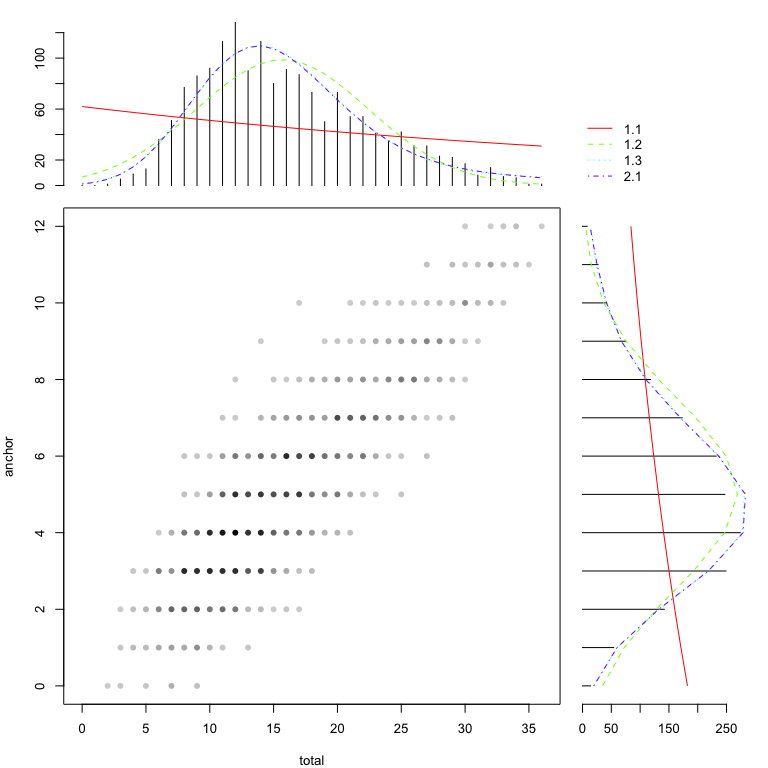Figure 5.4: Bivariate plot of KBneat total and anchor distributions with smoothed frequencies superimposed. round(rbind(x = summary(neat.x), xs = summary(neat.xs)), 2) #> mean sd skew kurt min max n #> x.total 15.82 6.53 0.58 2.72 2 36 1655 #> x.anchor 5.11 2.38 0.41 2.76 0 12 1655 #> xs.total 15.82 6.53 0.58 3.22 0 36 1655 #> xs.anchor 5.11 2.38 0.41 2.97 0 12 1655 The presmoothing function is used above to compare results from a sequence of nested models. The argument stepup = TRUE returns a matrix of fitted frequencies for models based on subsets of columns in scorefun, where the columns for each model can be specified with the argument models. The presmoothing function can also infer nested models when the degrees argument is used. In this case, terms are added sequentially for all variables within each level of interaction. For the example above, the first model in neat.xsmat includes the total and anchor scales to the first power, the second additionally includes both scales to the second power, and the third includes both to the third power. A fourth model contains the interaction term. The smoothed curves in the marginal distributions of Figure 5.4 show the loglinear smoothing results for each nested model that is fit in neat.xsmat. The legend text defines each smoothing curve using two numbers, the first for the level of interaction (1 for the first three models, and 2 for the fourth), and the second for the highest power included in a model (1, 2, and 3 for the main effects, and 1 for the interaction). Using the argument compare = TRUE in presmoothing, an ANOVA table of deviance statistics is returned for sequentially nested models. Model fit is compared using functions from the base packages in R (Team 2020), which provide $$AIC$$ (Akaike’s Information Criterion), $$BIC$$ (Bayesian Information Criterion), and likelihood ratio $$\chi^2$$ tests. In the output below, $$AIC$$ and $$BIC$$ are smallest for the most complex model, labeled Model 4, which also results in the largest decrease in deviance. The models being compared are the same as those contained in neat.xsmat. presmoothing(neat.x, smooth = "loglinear", degrees = list(c(3, 3), c(1, 1)), compare = TRUE) #> Analysis of Deviance Table #> #> Model 1: f ~ 1.0 + 0.1 #> Model 2: f ~ 1.0 + 0.1 + 2.0 + 0.2 #> Model 3: f ~ 1.0 + 0.1 + 2.0 + 0.2 + 3.0 + 0.3 #> Model 4: f ~ 1.0 + 0.1 + 2.0 + 0.2 + 3.0 + 0.3 + 1.1 #> Resid. Df Resid. Dev AIC BIC Df Deviance Pr(>Chi) CAIC CR #> 1 478 4574.1 5208.2 5220.8 4607.7 7338.2 #> 2 476 2699.7 3337.8 3358.7 2 1874.38 0.0000e+00 2750.1 6173.3 #> 3 474 2551.9 3194.1 3223.3 2 147.78 8.1255e-33 2619.2 5314.4 #> 4 473 333.8 977.9 1011.4 1 2218.12 0.0000e+00 409.5 2446.0 #> FT GM #> 1 4312.2 7.5095 #> 2 2437.2 3.6126 #> 3 2303.8 3.3054 #> 4 282.6 1.3061 Finally, with choose = TRUE, the presmoothing function will automatically select the best fitting model and return a smoothed frequency distribution based on that model. The deviance statistic for selection is indicated in the argument choosemethod, with options chi-square ("chi"), AIC ("aic"), and BIC ("bic"). For "aic" and "bic", the model with the smallest value is chosen. For "chi", the most complex model with $$p$$-value less than the argument chip is chosen, with default of $$1 - (1 - .05)^(1/(\# models - 1))$$. This automatic model selection is useful in simulation and resampling studies where unique presmoothing models must be fit at each replication. ## 5.4 The equate function Most of the functionality of the equate package can be accessed via the function equate, which integrates all of the equating types and methods introduced above. The equivalent-groups design provides a simple example: besides the $$X$$ and $$Y$$ frequency tables, only the equating type, i.e., the requested equating function, is required. equate(act.x, act.y, type = "mean") #> #> Mean Equating: act.x to act.y #> #> Design: equivalent groups #> #> Summary Statistics: #> mean sd skew kurt min max n #> x 19.85 8.21 0.38 2.30 1.00 40.00 4329 #> y 18.98 8.94 0.35 2.15 1.00 40.00 4152 #> yx 18.98 8.21 0.38 2.30 0.13 39.13 4329 #> #> Coefficients: #> intercept slope cx cy sx sy #> -0.8726 1.0000 20.0000 20.0000 40.0000 40.0000 The nonequivalent-groups design is specified with an equating method, and smoothing with a smoothmethod. neat.ef <- equate(neat.x, neat.y, type = "equip", method = "frequency estimation", smoothmethod = "log") Table 4.1 lists the equating methods that apply to each equating type in the nonequivalent-groups design. Levine true-score equating (lts) is performed by including the additional argument lts = TRUE. An equating object such as neat.ef contains basic information about the type, method, design, smoothing, and synthetic population weighting for the equating, in addition to the conversion table of equated scores and the original frequency distributions given for x and y. The summary method creates separate tables for all of the frequency distributions utilized in the equating, and calculates descriptive statistics for each one. summary(neat.ef) #> #> Frequency Estimation Equipercentile Equating: neat.x to neat.y #> #> Design: nonequivalent groups #> #> Smoothing Method: loglinear presmoothing #> #> Synthetic Weighting for x: 0.5025812 #> #> Summary Statistics: #> mean sd skew kurt min max n #> x.count 15.821 6.530 0.579 2.718 2.00 36.00 1655.000 #> x.smooth 15.821 6.530 0.579 2.718 0.00 36.00 1655.000 #> x.synth 16.726 6.761 0.438 2.456 0.00 36.00 1646.544 #> y.count 18.673 6.881 0.205 2.300 3.00 36.00 1638.000 #> y.smooth 18.673 6.881 0.205 2.300 0.00 36.00 1638.000 #> y.synth 17.742 6.805 0.338 2.413 0.00 36.00 1646.544 #> yx.obs 16.834 6.594 0.475 2.621 2.19 36.29 1655.000 #> xv.count 5.106 2.377 0.411 2.765 0.00 12.00 1655.000 #> xv.smooth 5.106 2.377 0.411 2.765 0.00 12.00 1655.000 #> xv.synth 5.481 2.444 0.259 2.565 0.00 12.00 1646.544 #> yv.count 5.863 2.452 0.107 2.507 0.00 12.00 1638.000 #> yv.smooth 5.863 2.452 0.107 2.507 0.00 12.00 1638.000 #> yv.synth 5.481 2.444 0.259 2.565 0.00 12.00 1646.544 The equate function can also be used to convert scores from one scale to another based on the function defined in a previous equating. For example, scores on $$Y$$ for a new sample of examinees taking KBneat form $$X$$ could be obtained. cbind(newx = c(3, 29, 8, 7, 13), yx = equate(c(3, 29, 8, 7, 13), y = neat.ef)) #> newx yx #> [1,] 3 3.276225 #> [2,] 29 29.814745 #> [3,] 8 8.696398 #> [4,] 7 7.614016 #> [5,] 13 14.125088 Here, the argument y passed to equate is the frequency estimation equipercentile equating object from above, which is an object of class equate. Since the equating function from neat.ef relates scores on $$X$$ to the scale of $$Y$$, anchor test scores are not needed for the examinees in newx. This feature provides a quick way to convert a score vector of any size from $$X$$ to $$Y$$. Because this feature does not rely on the discrete concordance table (i.e., conversion table) within the equating output, it can also be utilized with scores on $$X$$ that were not specified in the original equating, for example, non-integer values on $$X$$. The discrete concordance table can also be obtained. For some equating designs and methods, the concordance table will additionally include analytic standard errors. head(neat.ef$concordance)
#>   scale         yx
#> 1     0 0.04288325
#> 2     1 1.11109348
#> 3     2 2.18987042
#> 4     3 3.27622528
#> 5     4 4.36895117
#> 6     5 5.46596150

Finally, composite linkings are created using the composite function. For example, the identity and Tucker linear functions equating neat.x to neat.y could be combined as a weighted average.

neat.i <- equate(neat.x, neat.y, type = "ident")
neat.lt <- equate(neat.x, neat.y, type = "linear",
method = "tucker")
neat.comp <- composite(list(neat.i, neat.lt), wc = .5,
symmetric = TRUE)
plot(neat.comp, addident = FALSE)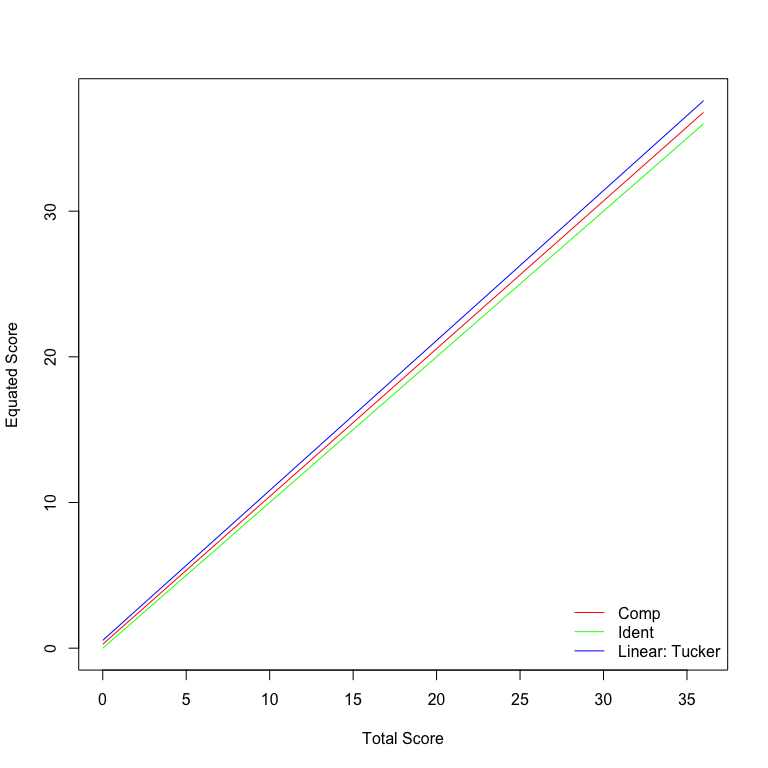Figure 5.5: Identity, Tucker linear, and a composite of the two functions for equating KBneat.

neat.comp represents what Kim, von Davier, and Haberman (2008) refer to as synthetic linear linking. The argument symmetric = TRUE is used to adjust the weighting system so that the resulting function is symmetric. Figure 5.5 shows the composite line in relation to the identity and linear components.

## 5.5 Linking with different scale lengths and item types

Procedures for linking scales of different lengths and item types are demonstrated here using PISA data. A frequency table containing four clusters, or item sets, from the PISA reading test was created above as pisa. This frequency table combines total scores on two item sets to create one form, R3R6, and total scores on two other item sets to create another form, R5R7. Because the same group of examinees took all of the item sets, the forms are contained within a single bivariate frequency table.

The two forms differ in length and item type. R3R6 contains 30 items, one of which has a maximum possible score of 2, and the remainder of which are scored dichotomously. This results in a score scale ranging from 0 to 31. However, 14 of the 30 items in R3R6 were multiple-choice, mostly with four response options. The remaining items were either constructed-response or complex multiple-choice, where examinees were unlikely to guess the correct response. Thus, the lowest score expected by chance for R3R6 is $$14/4 = 3.5$$. R5R7 contains 29 items, all of which are scored dichotomously. Eight of these items are multiple-choice with four response options and the remainder are constructed-response or complex multiple-choice, resulting in a lowest expected chance score of $$8/4 = 2$$. The summary statistics above show that, despite having a slightly smaller score scale, the mean for R5R7 is slightly higher than for R3R5.

Results for linking R3R6 to R5R7 are compared here for five linking types: identity, mean, linear, circle-arc, and equipercentile with loglinear presmoothing (using the default degrees}). By default, the identity linking component of each linear function is based on the minimum and maximum possible points for each scale, that is, $$(0, 0)$$ and $$(31, 29)$$. The low points were modified to be $$(3.5, 2)$$ to reflect the lowest scores expected by chance.

pisa.i <- equate(pisa, type = "ident", lowp = c(3.5, 2))
pisa.m <- equate(pisa, type = "mean", lowp = c(3.5, 2))
pisa.l <- equate(pisa, type = "linear", lowp = c(3.5, 2))
pisa.c <- equate(pisa, type = "circ", lowp = c(3.5, 2))
pisa.e <- equate(pisa, type = "equip", smooth = "log",
lowp = c(3.5, 2))
plot(pisa.i, pisa.m, pisa.l, pisa.c, pisa.e, addident = FALSE,
xpoints = pisa, morepars = list(ylim = c(0, 31)))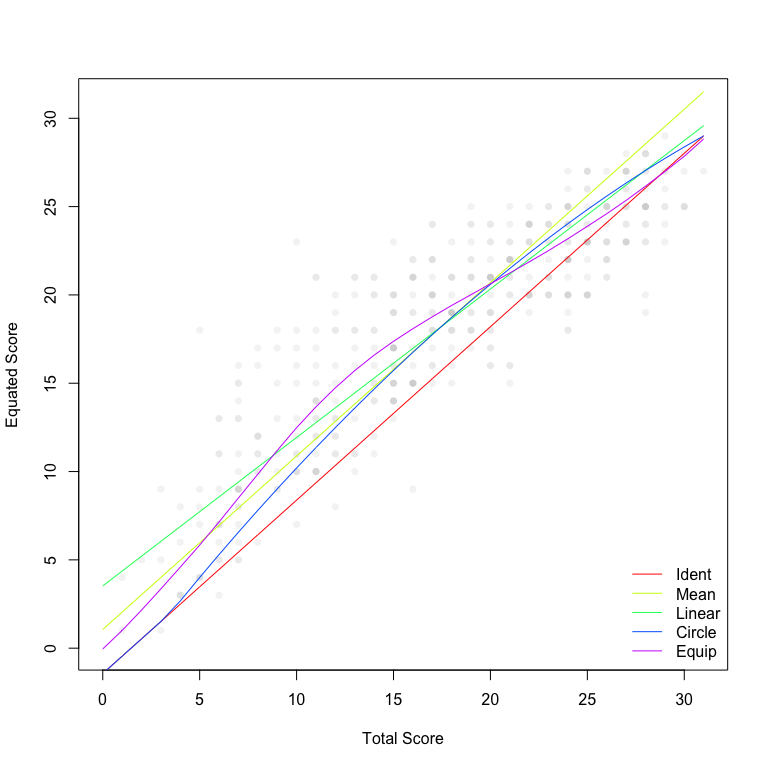Figure 5.6: Five functions linking R3R6 to R5R7.

The identity, mean, linear, circle-arc, and equipercentile linking functions are plotted in Figure 5.6. With a single-group design the linking lines can be plotted over the observed total scores for each form. In this way, the results can be compared in terms of how well each linking captures the observed difficulty difference from R3R6 to R5R7. Based on the scatterplot in Figure 5.6, scores on R5R7 tend to be higher, but this difference is not linear across the score scale. Instead, the difficulty difference appears curvilinear. Circle-arc linking appears to underestimate this nonlinearity, whereas equipercentile linking appears to estimate it well.

## 5.6 Linking with multiple anchors and covariates

The PISA data are used here to demonstrate linking with multiple anchor tests. As noted above, the PISA data come from a cluster rotation design, where different groups of students, organized by test booklet, saw different clusters of math, reading, and science items. Data from booklet 4 are used here to create two pseudo forms for a NEAT design with an external covariate. The unique reading items for each form come from clusters R3 and R4, the anchor comes from reading cluster R2, and the covariate from science cluster S2.

pisa.x <- freqtab(totals$b4[1:200, c("r3a", "r2", "s2")], scales = list(0:15, 0:17, 0:18)) pisa.y <- freqtab(totals$b4[201:400, c("r4a", "r2", "s2")],
scales = list(0:16, 0:17, 0:18))

Note that the first 200 students taking booklet 4 are used in pisa.x and the second 200 students are in pisa.y. One student in pisa.x had missing data and was excluded.

If multiple anchors and/or covariates are contained within a frequency table, they are automatically used by the equate function for any methods that support multi-anchor equating, and they are ignored for methods that do not support them. The following code conducts linking with covariates using the nominal weights, Tucker, and frequency estimation methods.

pisa.mnom <- equate(pisa.x, pisa.y, type = "mean",
method = "nom")
pisa.mtuck <- equate(pisa.x, pisa.y, type = "linear",
method = "tuck")
pisa.mfreq <- equate(pisa.x, pisa.y, type = "equip",
method = "freq", smooth = "loglin")

Single-anchor linking can also be performed by removing the science test from each frequency table. The margin function from the equate package is used here to extract only the bivariate distributions for the total and reading test anchor scales.

pisa.snom <- equate(margin(pisa.x, 1:2), margin(pisa.y, 1:2),
type = "mean", method = "nom")
pisa.stuck <- equate(margin(pisa.x, 1:2), margin(pisa.y, 1:2),
type = "linear", method = "tuck")
pisa.sfreq <- equate(margin(pisa.x, 1:2), margin(pisa.y, 1:2),
type = "equip", method = "freq", smooth = "loglin")

Figure 5.7, based on the code below, compares the single-anchor functions (solid lines) with the multi-anchor functions for each method (dashed lines).

plot(pisa.snom, pisa.stuck, pisa.sfreq,
pisa.mnom, pisa.mtuck, pisa.mfreq,
col = rep(rainbow(3), 2), lty = rep(1:2, each = 3))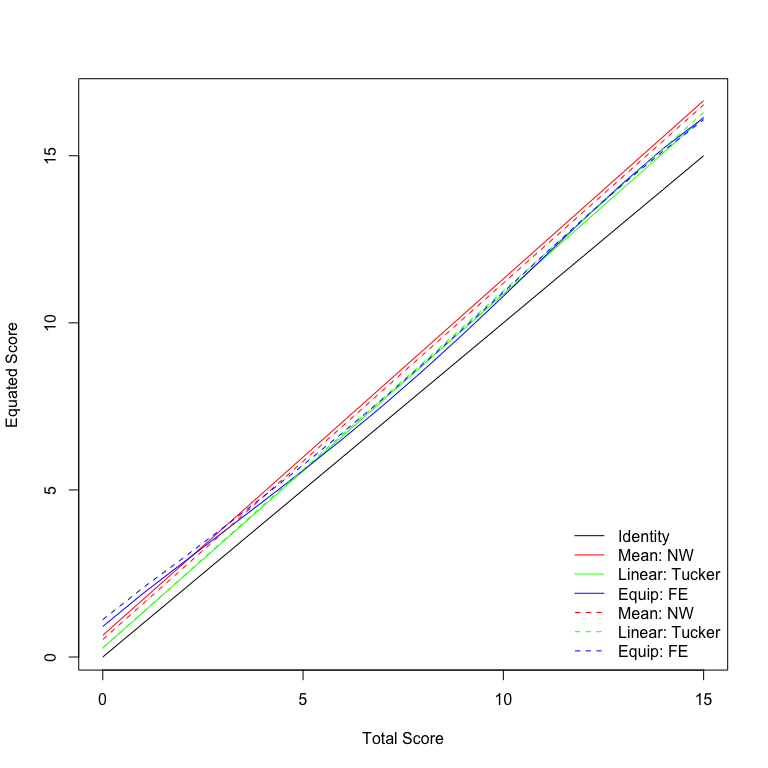Figure 5.7: Comparing single-anchor and covariate linking with PISA.

## 5.7 Bootstrapping

All but the identity linking and equating functions estimate a statistical relationship between score scales. Like any statistical estimate, equated scores are susceptible to bias and random sampling error, for example, as defined in Appendix 8.4. Standard error ($$SE$$), $$bias$$, and root mean square error ($$RMSE$$) can be estimated in the equate package using empirical and parametric bootstrapping.

With the argument boot = TRUE, the equate function will return bootstrap standard errors based on sample sizes of xn and yn taken across reps = 100 replications from x and y. Individuals are sampled with replacement, and the default sample sizes xn and yn will match those observed in x and y. Equating is performed at each replication, and the estimated equating functions are saved. $$Bias$$ and $$RMSE$$ can be obtained by including a vector of criterion equating scores via crit. Finally, the matrix of estimated equatings at each replication can be obtained with eqs = TRUE.

Parametric bootstrapping involves resampling as described above, but from a smoothed score distribution that is assumed to produce more reliable results with small samples (Kolen and Brennan 2014). In simulation studies this smoothed distribution is sometimes treated as a pseudo-population. Parametric bootstrapping is performed within the equate function by providing the optional frequency distributions xp and yp. These simply replace the sample distributions x and y when the bootstrap resampling is performed. Additionally, the bootstrap function can be used directly to perform multiple equatings at each bootstrap replication. $$SE$$, $$bias$$, and $$RMSE$$ can then be obtained for each equating function using the same bootstrap data.

Note that the number of bootstrap replications, specified via the reps argument, can impact the stability of the results, with error estimates varying noticeably for replications below 100. Bootstrapping studies vary widely in the number of replications utilized. It is recommended that no fewer than 100 be used. For more stable results, 500 to 1000 replications may be necessary, as computing time permits.

Parametric bootstrapping using the bootstrap function is demonstrated here for eight equatings of form $$X$$ to $$Y$$ in KBneat: Tucker and chained mean, Tucker and chained linear, frequency estimation and chained equipercentile, and Tucker and chained-linear circle-arc. Identity equating is also included. Smoothed population distributions are first created. Based on model fit comparisons, loglinear models were chosen to preserve 4 univariate and 2 bivariate moments in the smoothed distributions of $$X$$ and $$Y$$. Plots are shown in Figures 5.8 and 5.9.

neat.xp <- presmoothing(neat.x, "loglinear", degrees = list(4, 2))
neat.xpmat <- presmoothing(neat.x, "loglinear", degrees = list(4, 2),
stepup = TRUE)
neat.yp <- presmoothing(neat.y, "loglinear", degrees = list(4, 2))
neat.ypmat <- presmoothing(neat.y, "loglinear", degrees = list(4, 2),
stepup = TRUE)
plot(neat.x, neat.xpmat)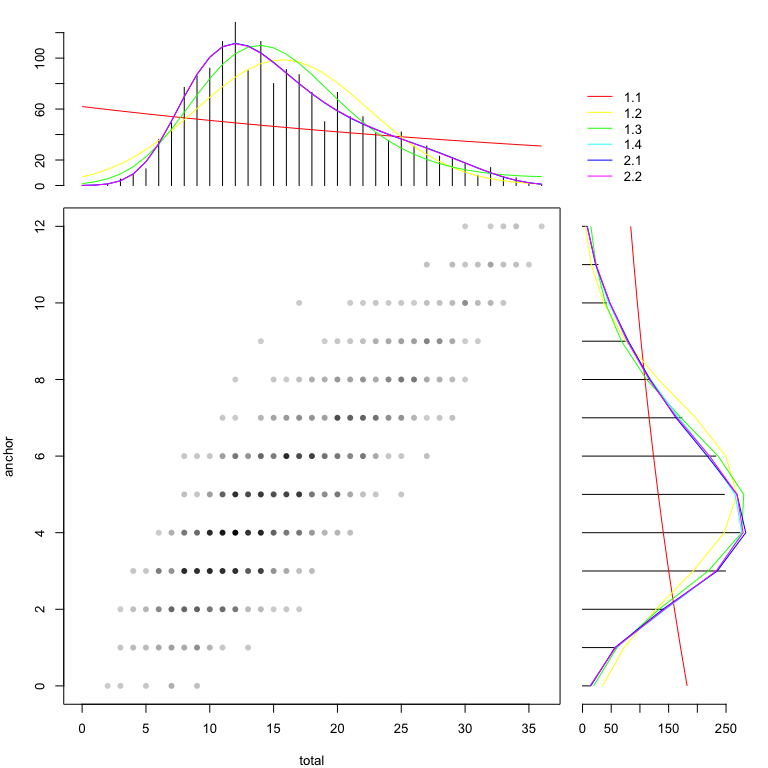Figure 5.8: Smoothed population distributions for $$X$$ used in parametric bootstrapping.

plot(neat.y, neat.ypmat)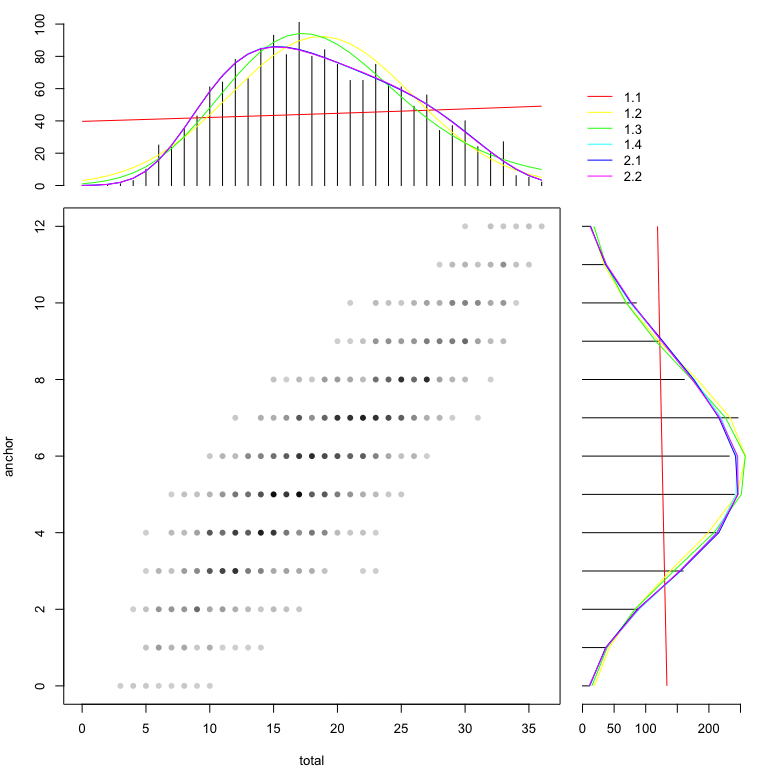Figure 5.9: Smoothed population distributions for $$Y$$ used in parametric bootstrapping.

Next, the number of replications is set to 100, bootstrap sample sizes are set to 100 for $$X$$ and $$Y$$, and a criterion equating function is defined, for demonstration purposes, as the chained equipercentile equating in the population.

set.seed(131031)
reps <- 100
xn <- 100
yn <- 100
crit <- equate(neat.xp, neat.yp, "e", "c")$conc$yx

Finally, to run multiple equatings in a single bootstrapping study, the arguments for each equating must be combined into a single object. Here, each element in neat.args is a named list of arguments for each equating. This object is then used in the bootstrap function, which carries out the bootstrapping.

neat.args <- list(i = list(type = "i"),
mt = list(type = "mean", method = "t"),
mc = list(type = "mean", method = "c"),
lt = list(type = "lin", method = "t"),
lc = list(type = "lin", method = "c"),
ef = list(type = "equip", method = "f", smooth = "log"),
ec = list(type = "equip", method = "c", smooth = "log"),
ct = list(type = "circ", method = "t"),
cc = list(type = "circ", method = "c", chainmidp = "lin"))
bootout <- bootstrap(x = neat.xp, y = neat.yp, xn = xn, yn = yn,
reps = reps, crit = crit, args = neat.args)

A plot method is available for visualizing output from the bootstrap function, as demonstrated below. Figures 5.10 through 5.13 contain the mean equated scores across replications for each method, the $$SE$$, $$bias$$, and $$RMSE$$. In Figure 5.10, the mean equated scores appear to be similar across much of the scale. Chained mean equating (the light orange line) consistently produces the highest mean equated scores. Mean equated scores for the remaining methods fall below those of chained mean and above those of identity equating (the black line). In Figure 5.11, standard errors tend to be highest for the equipercentile methods, especially chained equipercentile (the dark blue line), followed by the linear methods (green lines). $$SE$$ are lowest for the circle-arc methods (purple and pink), especially in the tails of the score scale where the identity function has more of an influence. In Figure 5.12, $$bias$$ is highest for chained mean equating, and is negative for the identity function; otherwise, $$bias$$ for the remaining methods falls roughly between -0.5 and 0.5. Finally, in Figure 5.13, $$RMSE$$ tends to be highest for chained mean and the linear and equipercentile methods. $$RMSE$$ for Tucker mean and the circle-arc methods tended to fall at or below 0.5.

plot(bootout, addident = FALSE, col = c(1, rainbow(8)))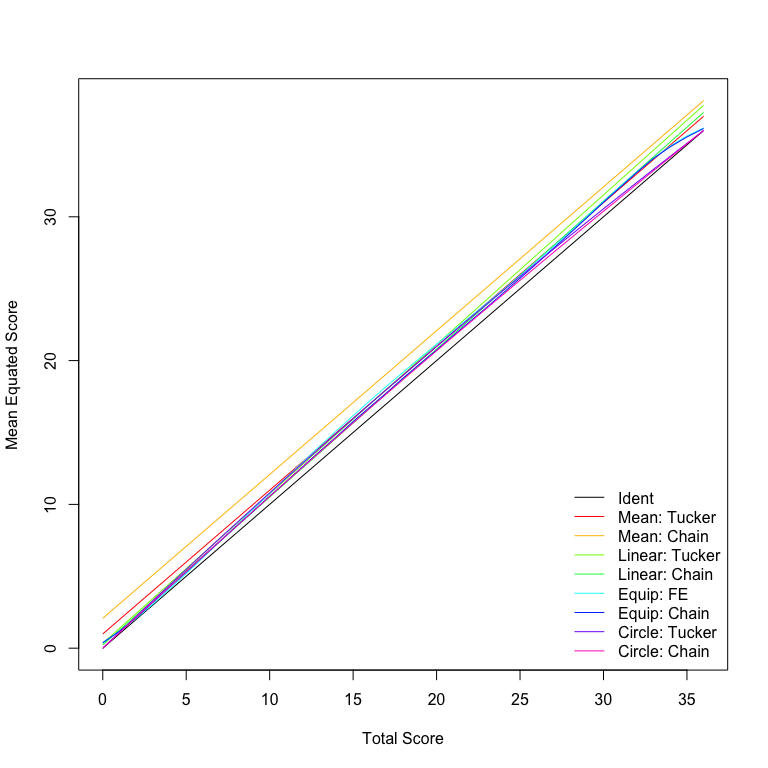Figure 5.10: Parametric bootstrapped mean equated scores for eight methods.

plot(bootout, out = "se", addident = FALSE,
col = c(1, rainbow(8)), legendplace = "top")